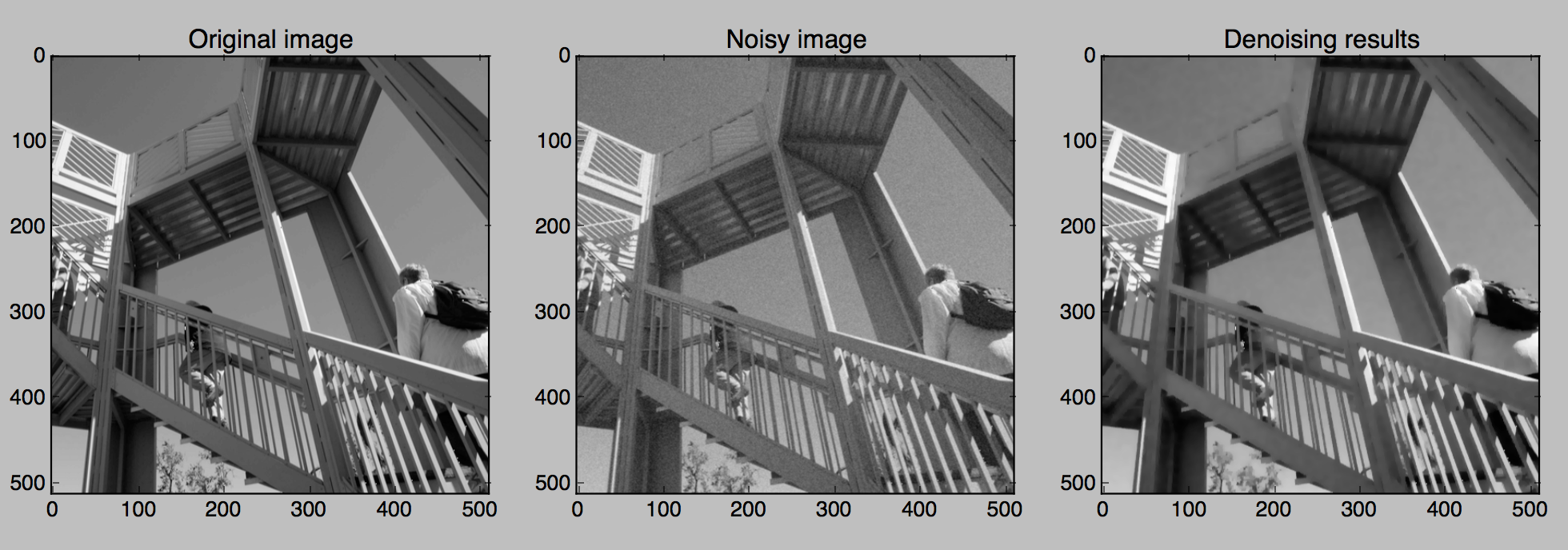# Welcome to ProxImaL¶

ProxImaL is a Python-embedded modeling language for image optimization problems. It allows you to express your problem in a natural way that follows the math, and automatically determines an efficient method for solving the problem. ProxImaL makes it easy to experiment with many different priors and other problem reformulations, without worrying about the details of how the problem is solved.

For example, the following code denoises an image using simple sparse gradient and nonnegativity priors:

from proximal import *
import numpy as np
import scipy.misc
import matplotlib.pyplot as plt

# Generate data.
I = scipy.misc.ascent()
np.random.seed(1)
b = I + 10*np.random.randn(*I.shape)

# Construct and solve problem.
x = Variable( I.shape )
prob = Problem(sum_squares(x - b/255) + .1*norm1( grad(x) ) + nonneg(x))
prob.solve()

# Plot the original, noisy, and denoised images.
plt.figure(figsize=(15,8))
plt.subplot(131)
plt.gray()
plt.imshow(I)
plt.title('Original image')

plt.subplot(132)
plt.gray()
plt.imshow(b)
plt.title('Noisy image')

plt.subplot(133)
plt.gray()
plt.imshow(x.value*255) # x.value is the optimal value of x.
plt.title('Denoising results')
plt.show()The example above uses simple, well-known priors. Much better results can be obtained using the more sophisticated priors provided in ProxImaL.

ProxImaL was designed and implemented by Felix Heide and Steven Diamond. See the accompanying paper for a full discussion of ProxImaL’s design and examples of state-of-the-art results obtained with ProxImaL.